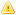Home |Sign in | EnglishHelpWLanguageWLanguage functionsStandard functionsNumeric values management functionsMatrix functionsMatExistPresentationExampleExistence of a matrix in memorySee alsoMatAddMatCopyMatCreateMatDeleteMatDeterminantMatErrorMatExistMatFillMatFloatAddMatFloatMultiplyMatInvertMatMultiplyMatNbColumnMatNbLineMatReadMatReadColumnMatReadLineMatStackMatTransposeMatWriteMatrix management functionsThe matricesThis content has been translated automatically. Click here to view the French version.
 Existence of a matrix in memory WINDEVWEBDEVWINDEV MobileOthersMatExist (Function) In french: MatExiste Checks the existence of a matrix in memory.Versions 25 and laterThis function is now available in browser code.New in version 25This function is now available in browser code.This function is now available in browser code.Example See additional examples // Teste l'existence de la matriceResExiste = MatExist("MaMatrice") Syntax = MatExist() : BooleanTrue if the matrix exists in memory,False otherwise. To get the details of the error, use ErrorInfo with the errMessage constant. : Character stringName of the matrix to be tested, defined with function MatCreate. Remarks Existence of a matrix in memoryA matrix can be created:by the function MatCreate,by copying a matrix (MatCopy),by adding two matrices (MatAdd),by multiplying two matrices (MatMultiply),by creating the transposed matrix (MatTranspose),by creating the matrix reverse( function MatInvert).A matrix can be deleted:by the function MatDelete,when closing the application.Business / UI classification: Neutral codeComponent: wd270mat.dll Minimum version required Version 9 This page is also available for…